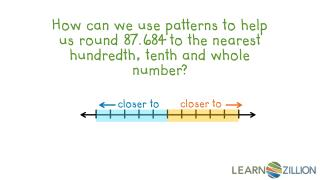# How can we use patterns to help us round 87.684 to the nearest hundredth, tenth and whole number? - PowerPoint PPT PresentationDownload PresentationHow can we use patterns to help us round 87.684 to the nearest hundredth, tenth and whole number?

How can we use patterns to help us round 87.684 to the nearest hundredth, tenth and whole number?Download Presentation## How can we use patterns to help us round 87.684 to the nearest hundredth, tenth and whole number?

- - - - - - - - - - - - - - - - - - - - - - - - - - - E N D - - - - - - - - - - - - - - - - - - - - - - - - - - -
##### Presentation Transcript

1. How can we use patterns to help us round 87.684 to the nearest hundredth, tenth and whole number? closer to closer to

2. In this lesson you will learn how to round decimals to any place by using patterns.

3. rounding \$36.87 \$40.00

4. 0.5 0.5 = 2.5 2 3

5. Misapplying a rounding “rule” in order to solve a problem. 4.48 4.5 5.0 4.48 4.0

6. Emily rounded some decimals to the nearest whole number. What patterns do you notice? If the tenths portion of the decimal is 0.5 or greater it rounds up. If the tenths portion of the decimal is 0.4 or less it rounds down. But why? closer to closer to 10 9 9.5

7. Andy rounded some decimals to the nearest tenth. What patterns do you notice? If the hundredths portion of the decimal is 0.05 or greater it rounds up. If the hundredths portion of the decimal is 0.04 or less it rounds down. But why? closer to closer to 6.40 6.30 6.35

8. If the thousandths portion of the decimal is 0.005 or greater it rounds up. If the thousandths portion of the decimal is 0.004 or less it rounds down. But why? Can we use the same patterns to help us round decimals to the nearest hundreth? closer to closer to 1.210 1.200 1.205

9. How can we use patterns to help us round 87.684 to the nearest hundredth, tenth and whole number? whole number hundredth tenth = 88 = 87.7 = 87.68 87.684 closer to closer to 87.69 87.7 88 87.68 87.6 87

10. How can we use patterns to help us round 87.684 to the nearest hundredth, tenth and whole number? 87.684 88 87.7 87.68 closer to closer to 87.69 88 87.7 87 87.68 87.6

11. In this lesson you have learned how to round decimals to any place by using patterns.